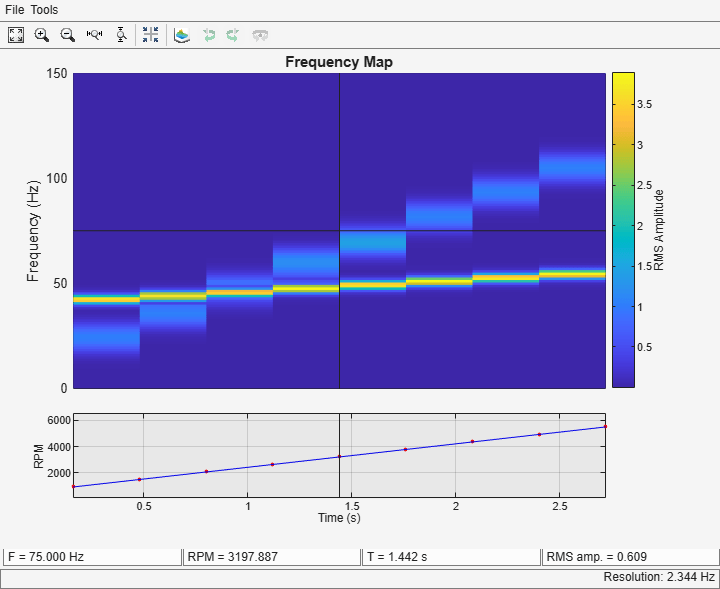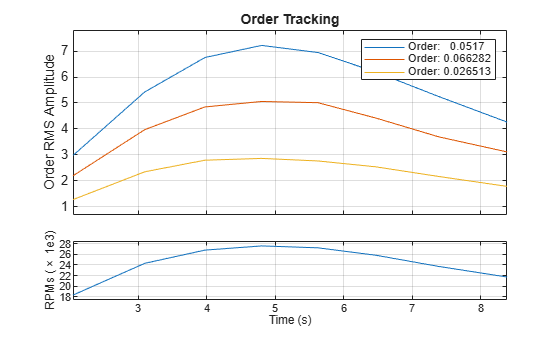# ordertrack

Track and extract order magnitudes from vibration signal

## Syntax

``mag = ordertrack(x,fs,rpm,orderlist)``
``````[mag,rpm,time] = ordertrack(x,fs,rpm,orderlist)``````
``[___] = ordertrack(x,fs,rpm,orderlist,rpmrefidx)``
``[___] = ordertrack(map,order,rpm,time,orderlist)``
``[___] = ordertrack(___,Name,Value)``
``ordertrack(___)``

## Description

example

````mag = ordertrack(x,fs,rpm,orderlist)` returns a matrix, `mag`, that contains time-dependent root-mean-square (RMS) amplitude estimates of a specified set of orders, `orderlist`, present in input signal `x`. `x` is measured at a set `rpm` of rotational speeds expressed in revolutions per minute. `fs` is the measurement sample rate in Hz.```
``````[mag,rpm,time] = ordertrack(x,fs,rpm,orderlist)``` also returns vectors of RPM and time values corresponding to the columns of `mag`.```
````[___] = ordertrack(x,fs,rpm,orderlist,rpmrefidx)` extracts order magnitudes using the first-order Vold-Kalman filter and returns any of the output arguments from previous syntaxes.```

example

````[___] = ordertrack(map,order,rpm,time,orderlist)` computes a matrix of magnitude estimates starting from an order-RPM map, `map`, a vector of orders, `order`, and a vector of time instants, `time`. Use `rpmordermap` to compute `map`, `order`, and `time`. The returned amplitudes and scaling are the same as in `map`.```

example

````[___] = ordertrack(___,Name,Value)` specifies further options using `Name,Value` pairs. Some of the options apply only to the Vold-Kalman tracking procedure.```
````ordertrack(___)` with no output arguments plots in the current figure the time-dependent orders and RPM values.```

## Examples

collapse all

Create a simulated signal sampled at 600 Hz for 5 seconds. The system that is being tested increases its rotational speed from 10 to 40 revolutions per second (or, equivalently, from 600 to 2400 revolutions per minute) during the observation period.

```fs = 600; t1 = 5; t = 0:1/fs:t1; f0 = 10; f1 = 40; rpm = 60*linspace(f0,f1,length(t));```

The signal consists of four harmonically related chirps with orders 1, 0.5, 4, and 6. The amplitudes of the chirps are 1, 1/2, √2, and 2, respectively. To generate the chirps, use the trapezoidal rule to express the phase as the integral of the rotational speed.

```o1 = 1; o2 = 0.5; o3 = 4; o4 = 6; a1 = 1; a2 = 0.5; a3 = sqrt(2); a4 = 2; ph = 2*pi*cumtrapz(rpm/60)/fs; x = [a1 a2 a3 a4]*cos([o1 o2 o3 o4]'*ph);```

Extract and visualize the magnitudes of the orders.

`ordertrack(x,fs,rpm,[o1 o2 o3 o4])`Create a simulated vibration signal consisting of two crossing orders corresponding to two different motors. The signal is sampled at 300 Hz for 3 seconds. The first motor increases its rotational speed from 10 to 100 revolutions per second (or, equivalently, from 600 to 6000 revolutions per minute) during the measurement. The second motor increases its rotational speed from 50 to 70 revolutions per second (or 3000 to 4200 revolutions per minute) during the same period.

```fs = 300; nsamp = 3*fs; rpm1 = linspace(10,100,nsamp)'*60; rpm2 = linspace(50,70,nsamp)'*60;```

The measured signal is of order 1.2 and amplitude 2√2 with respect to the first motor. With respect to the second motor, the signal is of order 0.8 and amplitude 4√2.

`x = [2 4]*sqrt(2).*cos(2*pi*cumtrapz([1.2*rpm1 0.8*rpm2]/60)/fs);`

Make the first motor excite a resonance at the middle of the frequency range.

```rs = [1+1./(1+linspace(-10,10,nsamp).^4)'/2 ones(nsamp,1)]; x = sum(rs.*x,2);```

Visualize the orders using `rpmfreqmap`.

`rpmfreqmap(x,fs,rpm1)`Compute the order magnitudes for both motors as a function of RPM. Use the Vold-Kalman algorithm to decouple the crossing orders.

`ordertrack(x,fs,[rpm1 rpm2],[1.2 0.8],[1 2],'Decouple',true)`Analyze simulated data from an accelerometer placed in the cockpit of a helicopter.

Load the helicopter data. The vibrational measurements, `vib`, are sampled at a rate of 500 Hz for 10 seconds. Inspection of the data reveals that it has a linear trend. Remove the trend to prevent it from degrading the quality of the order estimation.

```load('helidata.mat') vib = detrend(vib);```

Compute the order-RPM map. Specify an order resolution of 0.005.

`[map,order,rpm,time,res] = rpmordermap(vib,fs,rpm,0.005);`

Compute and plot the average order spectrum of the signal. Find the three highest peaks of the spectrum.

```[spectrum,specorder] = orderspectrum(map,order); [~,pkords] = findpeaks(spectrum,specorder,'SortStr','descend','Npeaks',3); findpeaks(spectrum,specorder,'SortStr','descend','Npeaks',3)```Track the amplitudes of the three highest peaks.

`ordertrack(map,order,rpm,time,pkords)`## Input Arguments

collapse all

Input signal, specified as a row or column vector.

Example: `cos(pi/4*(0:159))+randn(1,160)` specifies a sinusoid embedded in white Gaussian noise.

Data Types: `double` | `single`

Sample rate, specified as a positive scalar expressed in Hz.

Data Types: `double` | `single`

Rotational speeds, specified as a vector or matrix of positive values expressed in revolutions per minute. If `rpm` is a vector, it must have the same length as `x`. If `rpm` is a matrix, and `rpmrefidx` is specified, then `rpm` must have at least two columns, and each column must have as many elements as `x`.

• If you have a tachometer pulse signal, use `tachorpm` to extract `rpm` directly.

• If you do not have a tachometer pulse signal, use `rpmtrack` to extract `rpm` from a vibration signal.

Example: `100:10:3000` specifies that a system rotates initially at 100 revolutions per minute and runs up to 3000 revolutions per minute in increments of 10.

Data Types: `double` | `single`

List of orders, specified as a vector. `orderlist` must not have values larger than `fs`/(2 × max(`rpm`/60)).

Data Types: `double` | `single`

RPM column indices, specified as a vector of the same size as `orderlist`. The presence of this argument specifies that the Vold-Kalman algorithm is to be used.

Data Types: `double` | `single`

Order-RPM map, specified as a matrix. Use `rpmordermap` to compute order-RPM maps.

Data Types: `double` | `single`

Orders in order-RPM map syntax, specified as a vector. The length of `order` must equal the number of rows in `map`.

Data Types: `double` | `single`

### Name-Value Pair Arguments

Specify optional comma-separated pairs of `Name,Value` arguments. `Name` is the argument name and `Value` is the corresponding value. `Name` must appear inside quotes. You can specify several name and value pair arguments in any order as `Name1,Value1,...,NameN,ValueN`.

Example: `'Decouple',true,'Amplitude','peak'` extracts the specified orders simultaneously and returns the peak amplitude of each order.

Amplitude type, specified as the comma-separated pair consisting of `'Amplitude'` and one of `'rms'`, `'peak'`, or `'power'`.

• `'rms'` — Returns the root-mean-square amplitude for each estimated order.

• `'peak'` — Returns the peak amplitude for each estimated order.

• `'power'` — Returns the power level for each estimated order.

Magnitude scaling, specified as the comma-separated pair consisting of `'Scale'` and either `'linear'` or `'dB'`.

• `'linear'` — Returns magnitude values scaled in linear units.

• `'dB'` — Returns magnitude values scaled logarithmically and expressed in decibels.

Approximate half-power bandwidth, specified as the comma-separated pair consisting of `'Bandwidth'` and either a real scalar or a real vector with the same number of elements as `orderlist`. Smaller values of `'Bandwidth'` produce smooth, narrowband output. However, this output might not accurately reflect rapid changes in order amplitude. This argument applies only to the Vold-Kalman algorithm.

Data Types: `double` | `single`

Mode decoupling option, specified as the comma-separated pair consisting of `'Decouple'` and a logical value. If this option is set to `true`, then `ordertrack` extracts order magnitudes simultaneously, enabling it to separate closely spaced or crossing orders. This argument applies only to the Vold-Kalman algorithm.

Data Types: `logical`

Length of overlapping segments, specified as the comma-separated pair consisting of `'SegmentLength'` and an integer. If you specify a segment length, then `ordertrack` divides the input signal into segments. It then computes the order magnitudes for each segment and combines the results to produce the output. If the segments are too short, the function might not properly capture localized events such as crossing orders. This argument applies only to the Vold-Kalman algorithm.

Data Types: `double` | `single`

## Output Arguments

collapse all

Order-magnitude matrix, returned as a matrix.

Rotational speeds, returned as a vector of positive values expressed in revolutions per minute.

Time instants, returned as a vector.

 Brandt, Anders. Noise and Vibration Analysis: Signal Analysis and Experimental Procedures. Chichester, UK: John Wiley & Sons, 2011.

 Feldbauer, Christian, and Robert Höldrich. "Realization of a Vold-Kalman Tracking Filter — A Least Squares Problem." Proceedings of the COST G-6 Conference on Digital Audio Effects (DAFX-00). Verona, Italy, December 7–9, 2000.

 Vold, Håvard, and Jan Leuridan. "High Resolution Order Tracking at Extreme Slew Rates Using Kalman Tracking Filters." Shock and Vibration. Vol. 2, 1995, pp. 507–515.

 Tůma, Jiří. “Algorithms for the Vold-Kalman Multiorder Tracking Filter.” Proceedings of the 14th International Carpathian Control Conference (ICCC), 2013, pp. 388–94. https://doi.org/10.1109/CarpathianCC.2013.6560575.